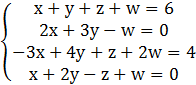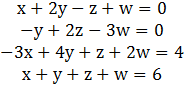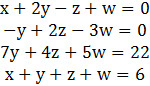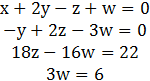### Sample Problem

Solve using the elimination method:x=

y=

z=

w=

#### SolutionSwitch  E4 with E1E2=E2-2E1E3=E3+3E4E4=E4-1E1

E3=E3+7E2E4=E4-1E2E2*-1=E2

3/18=E3

E4/3=E4Now back substitute w=2 into the 3rd equation to solve for z

z-8/9w=11/9

z=11/9+8/9w

z=11/9+(8/9)(2)=11/9+16/9=27/9=3

Now back substitute w=2 and z=3 into the 2nd equation to solve for y

y-2z+3w=0

y=-3w+2z

y=-3(2)+2(3)=0

Now back substitute w=2, z=3, and y=0 into the 1st equation to solve for x

x+2y-z+w=0

x=-w+z-2y=-(2)+(3)-2(0)=-2+3=1

x=1 ; y=0 ; z=3 ; w=2